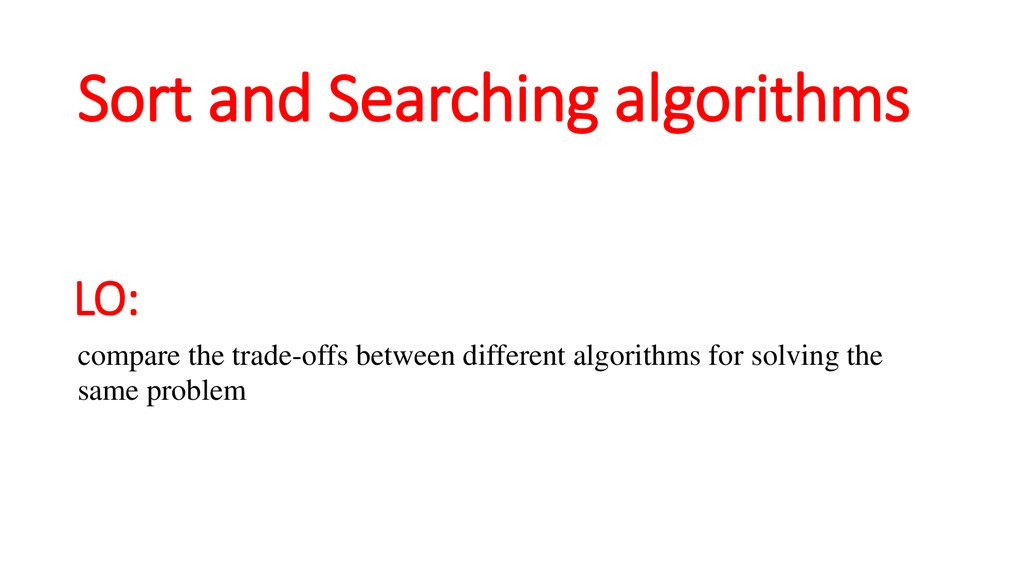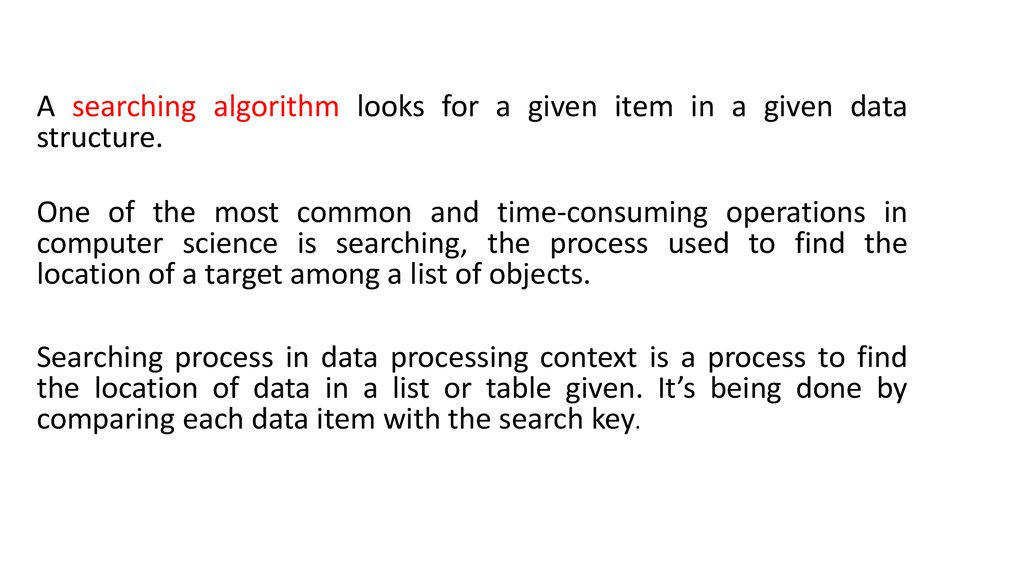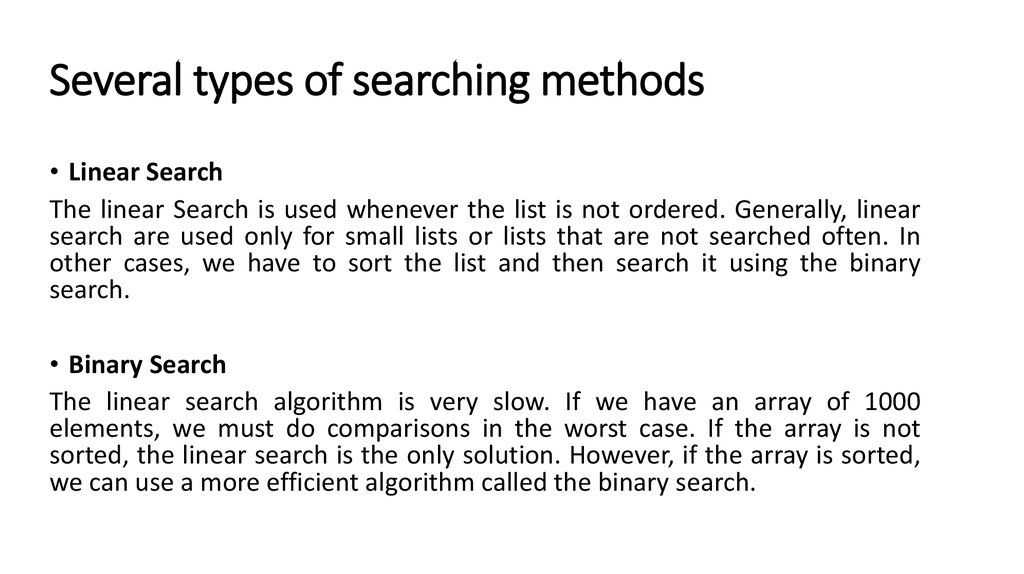# Sort and Searching algorithms

## 1. Sort and Searching algorithms

LO:
compare the trade-offs between different algorithms for solving the
same problem

## 2.

A searching algorithm looks for a given item in a given data
structure.
One of the most common and time-consuming operations in
computer science is searching, the process used to find the
location of a target among a list of objects.
Searching process in data processing context is a process to find
the location of data in a list or table given. It’s being done by
comparing each data item with the search key.

## 3. Several types of searching methods

• Linear Search
The linear Search is used whenever the list is not ordered. Generally, linear
search are used only for small lists or lists that are not searched often. In
other cases, we have to sort the list and then search it using the binary
search.
• Binary Search
The linear search algorithm is very slow. If we have an array of 1000
elements, we must do comparisons in the worst case. If the array is not
sorted, the linear search is the only solution. However, if the array is sorted,
we can use a more efficient algorithm called the binary search.

## 4. Sort algorithms

A sorting algorithm is an algorithm that puts elements of a list in a certain
order. The most-used orders are numerical order and lexicographical order.
Sorting is any process of arranging items in some sequence and/or in
different sets, and accordingly, it has two common, yet distinct meanings:
1. Ordering: arranging items of the same kind, class, nature, etc. in some
ordered sequence
2. Categorizing: grouping and labelling items with similar properties
together (by sorts)

## 5. Several types of sorting methods

Selection Sort
Selection sort is a sorting algorithm, specifically an in-place comparison sort. Selection sort is noted for its
simplicity, and also has performance advantages over more complicated algorithms in certain situations.
Insertion Sort
Insertion sort is a simple sorting algorithm, a comparison sort in which the sorted array (or list) is built one
entry at a time. It is much less efficient on large lists than more advanced algorithms.
Bubble Sort
Bubble sort is a simple sorting algorithm. It works by repeatedly stepping through the list to be sorted,
comparing two items at a time and swapping them if they are in the wrong order. The pass through the list is
repeated until no swaps are needed, which indicates that the list is sorted. The algorithm gets its name from
the way smaller elements "bubble" to the top of the list. Because it only uses comparisons to operate on
elements, it is a comparison sort.
Merge Sort
Merge sort is a comparison-based sorting algorithm. In most implementations it is stable, meaning that it
preserves the input order of equal elements in the sorted output. It is an example of the divide and conquer
algorithmic paradigm. It was invented by John von Neumann in 1945.
Quick Sort
As one of the more advanced sorting algorithms, you might think that the Quick sort Algorithm is steeped in
complicated theoretical background, but this is not so. Like Insertion Sort, this algorithm has a fairly simple
concept at the core, but is made complicated by the constraints of the array structure.

## 6.

• Q1. What is Sorting and Searching algorithms?
• Q2. List down 3 methods of sorting
• Q3. List down 3 types of searching method

## 7. Summary

• One of the most common applications in computer science is sorting.
• Data may be sorted in either ascending or descending order.
• There are several types of sorting such as selection, insertion, bubble,
merge and quick sort.
• Searching is the process of finding the location of a target among list of
objects.
• There are several types of searching methods such as linear, binary.

## 9. Learning objectives

• write a binary search algorithm to solve a particular
problem
• show understanding of the conditions necessary for the
use of a binary search

## 10. Linear Search

Linear search is a very simple search algorithm. In this
type of search, a sequential search is made over all
items one by one. Every item is checked and if a match
is found then that particular item is returned,
otherwise the search continues till the end of the data
collection.

## 12. Binary Search

This search algorithm works on the principle of divide and conquer. For this
algorithm to work properly, the data collection should be in the sorted form.
Binary search looks for a particular item by comparing the middle most item
of the collection. If a match occurs, then the index of item is returned. If the
middle item is greater than the item, then the item is searched in the subarray to the left of the middle item. Otherwise, the item is searched for in the
sub-array to the right of the middle item. This process continues on the subarray as well until the size of the subarray reduces to zero.

Binary Search